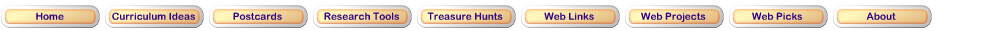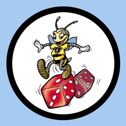NCTM Data Analysis and Probability Standard for Grades 3–5 Understand and apply basic concepts of probability • predict the probability of outcomes of simple experiments and test the predictions; Step 1Read this introduction that explains probability.Step 2Ready to try an experiment with probability? 1. Go to Illuminations. 2. Choose 6 colors. 3. Predict how many times the spinner will land on each color. 4. Click on Record Results. 5. Type in 100 spins. 6. Click on Spin. check the online results and record them in the Spin Experiment spreadsheet. 7. What does the graph tell you about the likelihood of the spinner landing on each color? 8. Type in 500 spins. 9. Click on Spin. 10. Graph the results. 11. What does the graph tell you about the likelihood of the spinner landing on each color? 12. How did the two experiments compare?Updated March 14, 2017
© 1996 - 2015 Linda C. Joseph